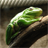# QlikView App Dev

Discussion Board for collaboration related to QlikView App Development.

Announcements
cancel
Showing results for
Did you mean:Not applicable

## Using a variable in set analysis

I have set a Current Month variable:

vCurrentMonth= Month(Today(2));

Which works in a text object, it shows "Jun" which is what I am looking for.

The expression I use the variable in is as follows:

=Count( {\$< EndMonth =  { '\$(vCurrentMonth)' } >} [Assignment ID] )

Which results in zeros. But if I type Jun in place of the variable I get the correct data.

I've tried:

=Count( {\$< EndMonth = { '=\$(vCurrentMonth)' } >} [Assignment ID] )        Shows all data as if there was no set analysis

=Count( {\$< EndMonth = { ='\$(vCurrentMonth)' } >} [Assignment ID] )        null values

=Count( {\$< EndMonth = { \$('vCurrentMonth') } >} [Assignment ID] )          null values

=Count( {\$< EndMonth = { "\$(vCurrentMonth)" } >} [Assignment ID] )         null values

I'm all out of ideas for why this isn't working. Can anyone see a mistake in the syntax or have any insight for why this variable is not working.

Thanks,

1 Solution

Accepted SolutionsSpecialist II

You'll want to use the keyword LET in your script:

LET vCurrentMonth= Month(Today(2));

This will put the value 'Jun" into your variable. Then your formula:

=Count( {\$< EndMonth =  {\$(vCurrentMonth)} >} [Assignment ID] )

will work. Then you can just have the formula for your textbox be:

=vCurrentMonth

Hope this helps!

7 RepliesSpecialist II

try:

Count( {\$< EndMonth = { '\$(=vCurrentMonth)' } >} [Assignment ID] )

Hope this helps!MVP

this

=count({\$ <Month={'\$(vCurrentMonth)'}>} [Assignment ID] )

and this

=count({\$ <Month={\$(vCurrentMonth)}>} [Assignment ID] )

should work

=Month(Today(2))

or

Month(Today(2))

?Specialist II

Here's another one you can try:

Count( {\$< EndMonth = {'\$(=\$(vCurrentMonth)) } >} [Assignment ID] )

As Massimo Grossi brings up, having or not having the equal sign in the declaration of the variable will affect your expression. The above should work if it does not have the equal sign. The others listed should work if it has the equal signNot applicable
Author

The variable does not include the equal sign.

The script: SET vCurrentMonth= Month(Today(2));

Is it best practice to include the equal sign as well?

example: SET vCurrentMonth= =Month(Today(2));Not applicable
Author

Your answer works and, as you said, Massimo's answer works if I change the variable to have an equal sign.

SET vCurrentMonth= =Month(Today(2));

However once I add the value sign my text object no longer displays the data.

Expression: =\$(vCurrentMonth)

It used to display Jun, now it produces a null value "-"Specialist II

You'll want to use the keyword LET in your script:

LET vCurrentMonth= Month(Today(2));

This will put the value 'Jun" into your variable. Then your formula:

=Count( {\$< EndMonth =  {\$(vCurrentMonth)} >} [Assignment ID] )

will work. Then you can just have the formula for your textbox be:

=vCurrentMonth

Hope this helps!Creator III

Super Awesome. I was so baffled as to why my text entry was not working lol. Thanks for the help